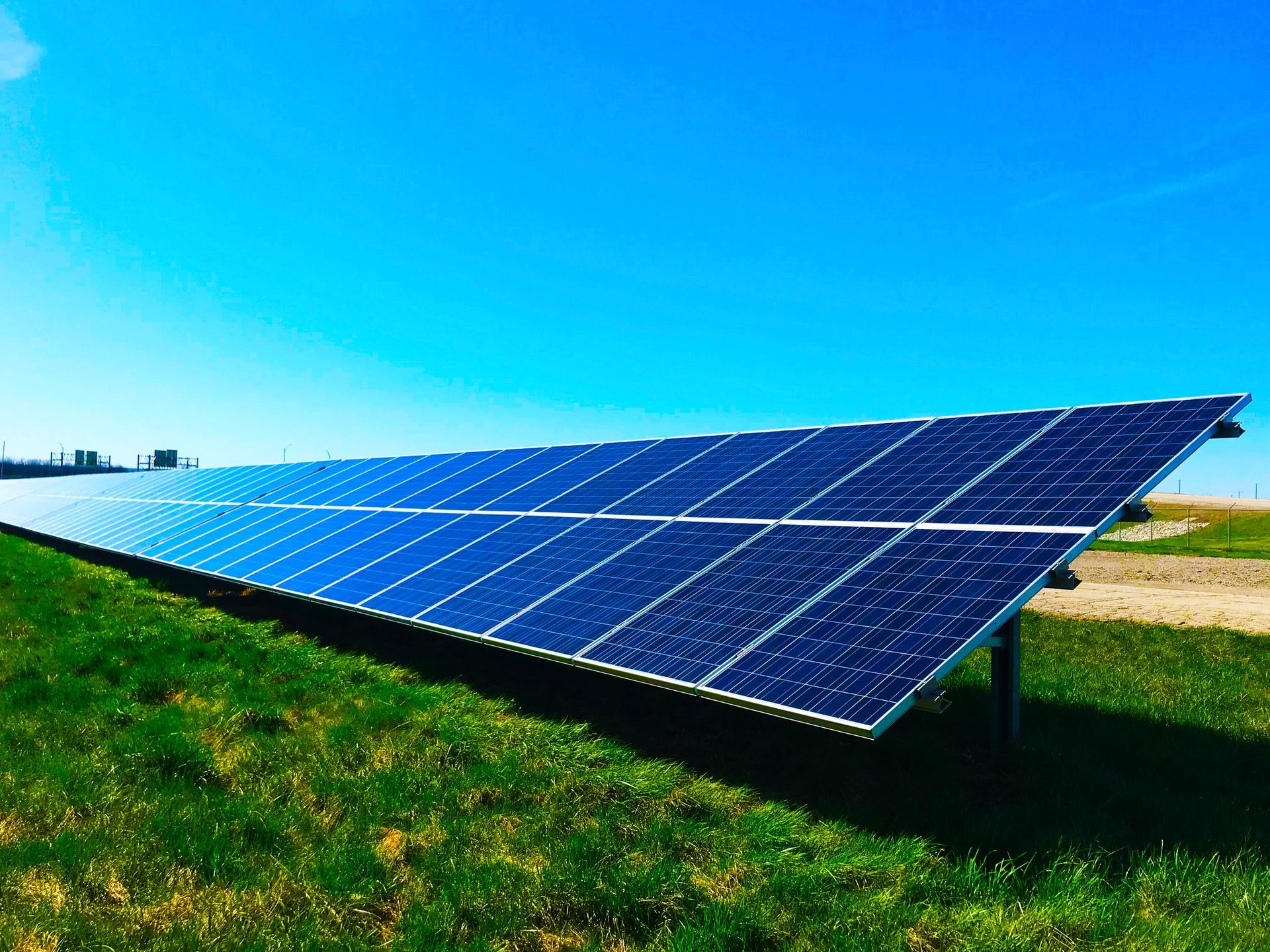# Python Code: Technical cost-benefit analysis of a PV system complemented with energy storage for increased electricity self-sufficiency.

## Description and step-wise instructions on how to use the Python code

The analysis was conducted in Python and the corresponding scripts and data can be downloaded by following the link above. The scripts are flexible so that different scenarios in terms of consumption, PV generation, and energy storage capacity can be analyzed. All parameters that are shown in Table 1 in “Photovoltaic (PV) and energy storage: a viable symbiosis or an overrated investment?” can be adjusted.

The Python code for the analysis is divided into three scripts. Only one additional data file (ESO_synthload2021.xlsx) with the yearly load and PV profiles is needed. This file is delivered along with the code. In the following, you find a stepwise description on how to use the three scripts and what’s happening in each of them.

Note: of course you can also plug in your own load and PV profiles. Just make sure that they have the same format as the ones in ‘ESO_data_load_PV_pu.pkl’ which are the result of the data pre-processing. Remember that the profiles represent the per unit energy consumption for each time unit over the whole year. Accordingly, the sum of each per unit profile is equal to one.

#### How?

• Simply run the script if you keep the file ‘ESO_synthload2021.xlsx’ in the same folder as the script, no adjustments needed.
• If you keep ‘ESO_synthload2021.xlsx’ in a different folder, add the path as shown in Screenshot 1 before you run the script.

#### What?

• Resamples the consumption and PV profile from quarter-hourly to hourly resolution.
• Rescales the data to per unit, i.e. the sum of the consumption and PV profile over one year is equal to one, thus the profile can be easily scaled with the yearly consumption and PV generation, respectively.
• Saves the profiles as ‘ESO_data_load_PV_pu.pkl’.

### Script 2: ESO_02_energy_storage_optimization_full_year.py

#### How?

• Define the parameters for the calculation as shown in Screenshot 2.
• Storage
• spec_eta: efficiency (pu)
• spec_Emin: min. energy content (kWh)
• spec_E0: initial energy content (kWh) must be greater or equal than the min. energy content.
• spec_P_minmax_scale: min./max. power rating (pu) based on the storage capacity, Pmin/Pmax = -/+ spec_P_minmax_scale * storage capacity
• Consumption and specific PV yield (yearly)
• PV_spec_yield: specific yearly PV yield (kWh/kWp) defining how much energy per installed kWp is produced per year
• PV and energy storage range for the analysis
• PV_from, PV_to, PV_step: min. PV, max. PV, step (kWp) defining the minimum, maximum, and step size for the PV system. Example: PV_from, PV_to, PV_step = 6, 18, 6 means from 6 to 18 kW in 6 kW steps.
• E_from, E_to, E_step: min., max. storage capacity, step (kWh) defining the minimum, maximum, and step size for the storage capacity. Example: E_from, E_to, E_step = 0, 15, 3 means from 0 to 15 kWh in 3 kWh steps. Recommendation: always start from 0 kWh because the savings are calculated based on these results.
• Run the script.

#### What?

• Scales the consumption and PV profile with the specified yearly consumption and PV yield, respectively.
• Calculates the optimal energy storage schedule by minimizing the exchange with the grid based on the yearly consumption and PV profiles. The optimization takes care of ensuring that the storage system specifications are satisfied at all points in time, e.g. max. capacity.
• Repeats the calculation for all specified energy storage and PV capacities.
• Saves a result file for each PV capacity containing the consumption profile, PV profile, and optimal storage schedule for the whole year. Each file name includes the most relevant parameters of the calculation, e.g. yearly load, PV capacity, etc. Below two example result files are shown (Load = 3000 kWh, PV = 6, 12 and 18 kWp, Emax = 0 – 15 kWh in 3 kWh steps, eta = 0.9 and E0 = 0 kWh). Do not change the name of the result files as some parameters are extracted from the filename in the next step.

### Script 3: ESO_03_result_analysis.py

#### How?

• Define the parameters as shown in Screenshot 4.
• tariff_el: Electricity tariff (€/kWh)
• tariff_PV: Remuneration for PV generation (€/kWh)
• cost_storage_per_kWh: specific costs of the storage system (€/kWh)
• Run the script

#### What?

• Calculates the exchanged energy based on the consumption profile, PV profile, and optimal energy storage schedule. The exchanged energy is divided into two groups: energy consumed from the grid and energy supplied to the grid.
• Calculates the costs for the consumed energy according to the electricity tariff and the remuneration from PV surplus according to the PV tariff. The sum of these two positions yields the yearly electricity costs.
• Calculates the total costs which are comprised of the electricity costs and the storage costs.
• Calculates the yearly savings based on the zero storage scenario for each PV case.
• Calculates the amortization period based on the yearly savings without storage costs and the total storage costs.
• Prints a summary of the results in the console as shown in the screenshot below. The summary shows for each PV scenario the optimal storage capacity based on the maximum savings. Additionally, the costs and amortization period at the optimal storage capacity is shown.
• Plots three plots showing the yearly costs, savings, and amortization period. Remember that the costs and savings include the storage costs. The plots are the same as in my study case (Fig. 2 – 4).